Find Paper, Faster
Example：10.1021/acsami.1c06204 or Chem. Rev., 2007, 107, 2411-2502
PERTURBATION THEOREMS FOR FRACTIONAL CRITICAL EQUATIONS ON BOUNDED DOMAINS
Journal of the Australian Mathematical Society  (IF0.8),  Pub Date : 2020-03-09, DOI: 10.1017/s144678871900048x
AZEB ALGHANEMI, HICHEM CHTIOUI

We consider the fractional critical problem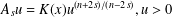$A_{s}u=K(x)u^{(n+2s)/(n-2s)},u>0$ in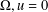$\unicode[STIX]{x1D6FA},u=0$ on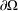$\unicode[STIX]{x2202}\unicode[STIX]{x1D6FA}$ , where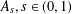$A_{s},s\in (0,1)$ , is the fractional Laplace operator and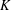$K$ is a given function on a bounded domain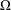$\unicode[STIX]{x1D6FA}$ of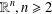$\mathbb{R}^{n},n\geq 2$ . This is based on A. Bahri’s theory of critical points at infinity in Bahri [Critical Points at Infinity in Some Variational Problems, Pitman Research Notes in Mathematics Series, 182 (Longman Scientific & Technical, Harlow, 1989)]. We prove Bahri’s estimates in the fractional setting and we provide existence theorems for the problem when$K$ is close to 1.